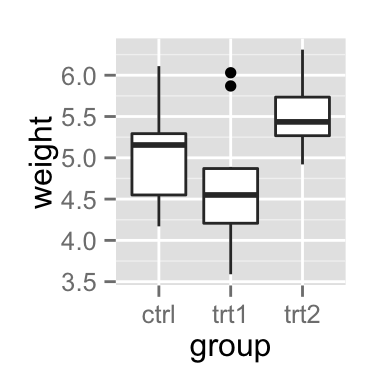# ggplot2 rotate a graph : reverse and flip the plot

The aim of this R tutorial is to describe how to rotate a plot created using R software and ggplot2 package.

The functions are :

• coord_flip() to create horizontal plots
• scale_x_reverse(), scale_y_reverse() to reverse the axes

# Horizontal plot : coord_flip()

Box plot :

``````library(ggplot2)
# Basic box plot
bp <- ggplot(PlantGrowth, aes(x=group, y=weight))+
geom_boxplot()
bp
# Horizontal box plot
bp + coord_flip()``````Histogram :

``````set.seed(1234)
# Basic histogram
hp <-qplot(x=rnorm(200), geom="histogram")
hp
# Horizontal histogram
hp + coord_flip()``````# Reverse y axis

The function scale_y_reverse() can be used as follow :

``````# Basic histogram
hp
# Y axis reversed
hp + scale_y_reverse()``````# Infos

This analysis has been performed using R software (ver. 3.1.2) and ggplot2 (ver. )